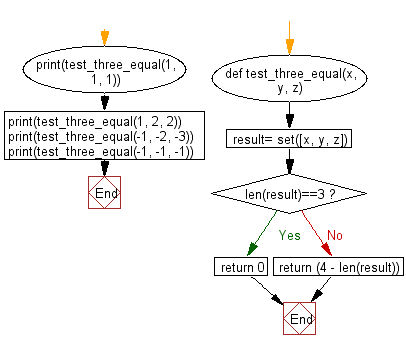﻿ Python: Count the number of equal numbers from three given integers - w3resource

# Python: Count the number of equal numbers from three given integers

## Python Basic - 1: Exercise-86 with Solution

Write a Python program to count the number of equal numbers from three given integers.

Sample Solution:

Python Code:

``````def test_three_equal(x, y, z):
result= set([x, y, z])
if len(result)==3:
return 0
else:
return (4 - len(result))

print(test_three_equal(1, 1, 1))
print(test_three_equal(1, 2, 2))
print(test_three_equal(-1, -2, -3))
print(test_three_equal(-1, -1, -1))
``````

Sample Output:

```3
2
0
3
```

Pictorial Presentation:Flowchart:Python Code Editor:

Have another way to solve this solution? Contribute your code (and comments) through Disqus.

What is the difficulty level of this exercise?

Test your Programming skills with w3resource's quiz.

﻿